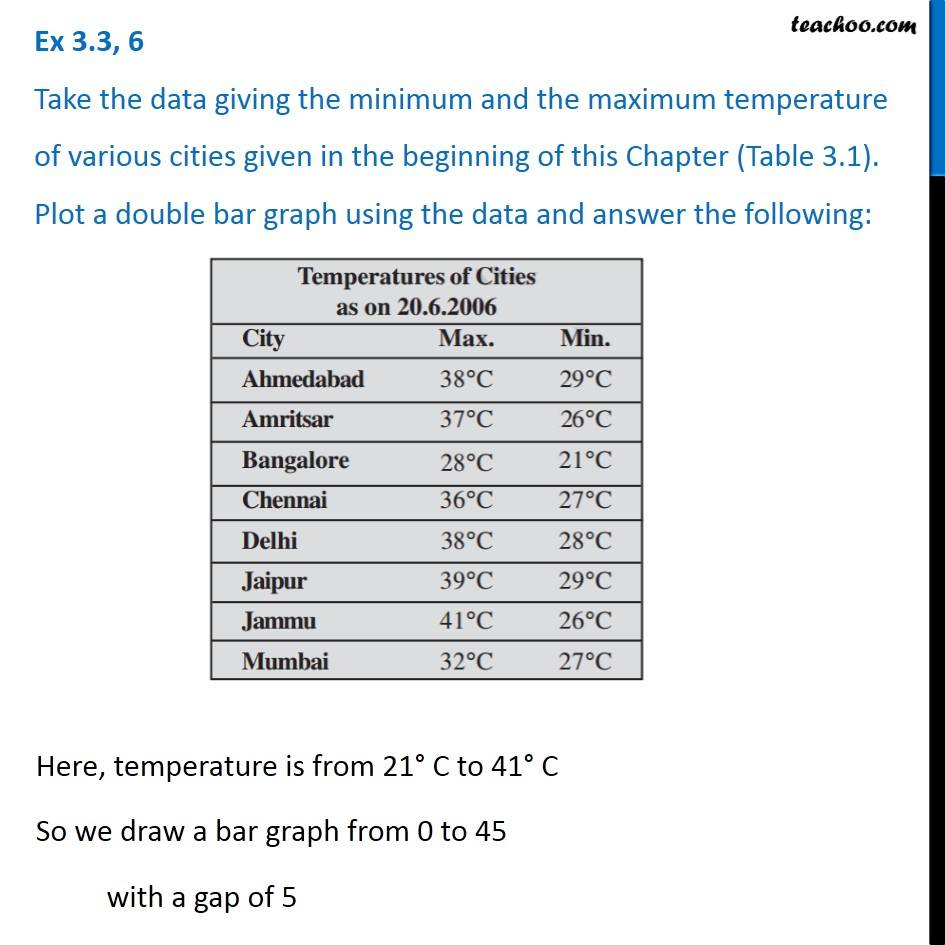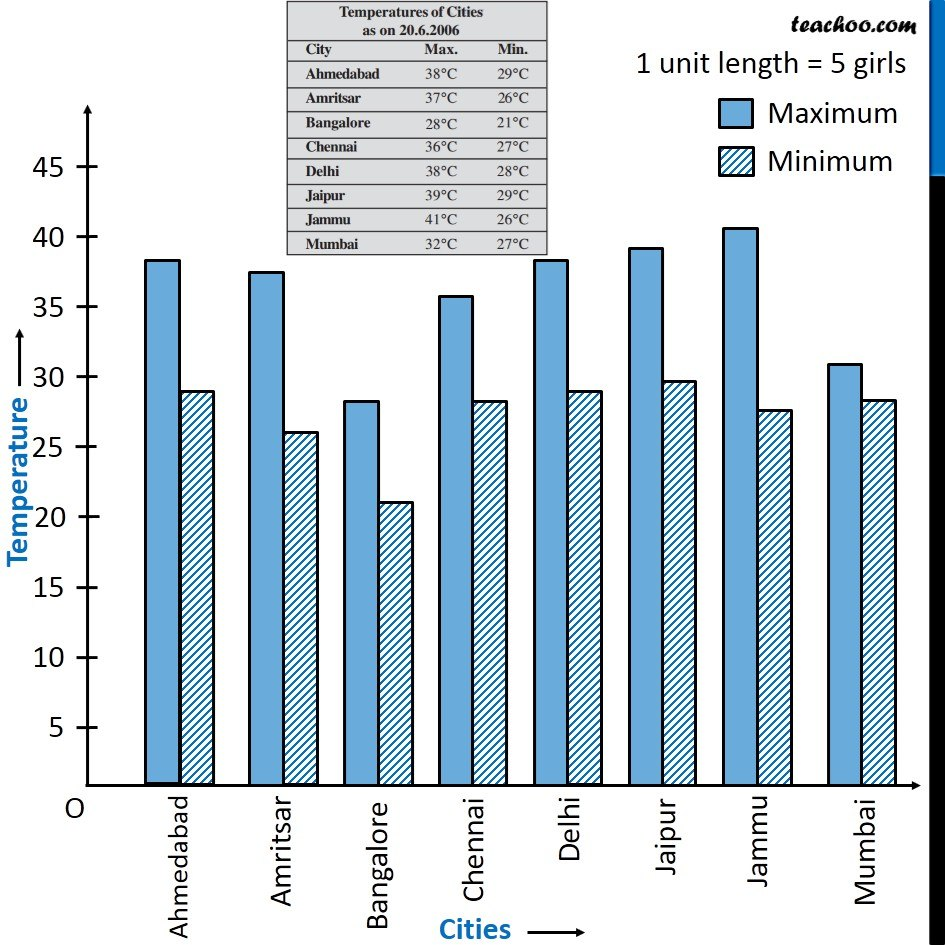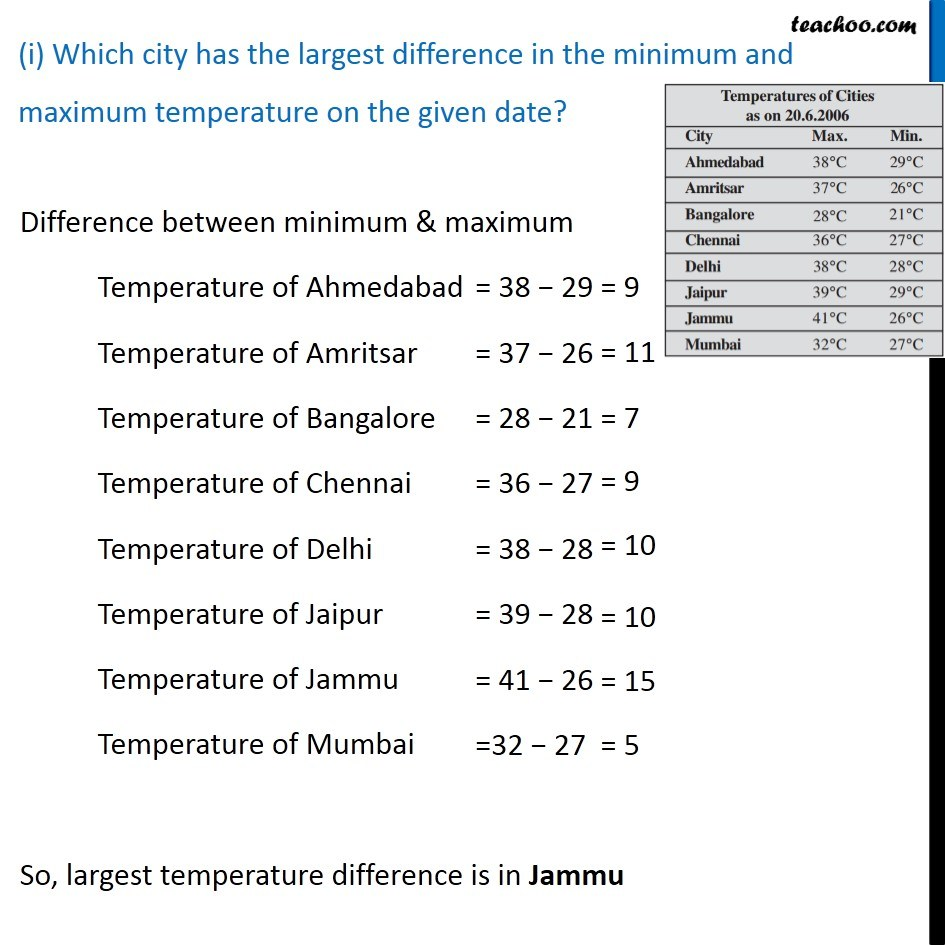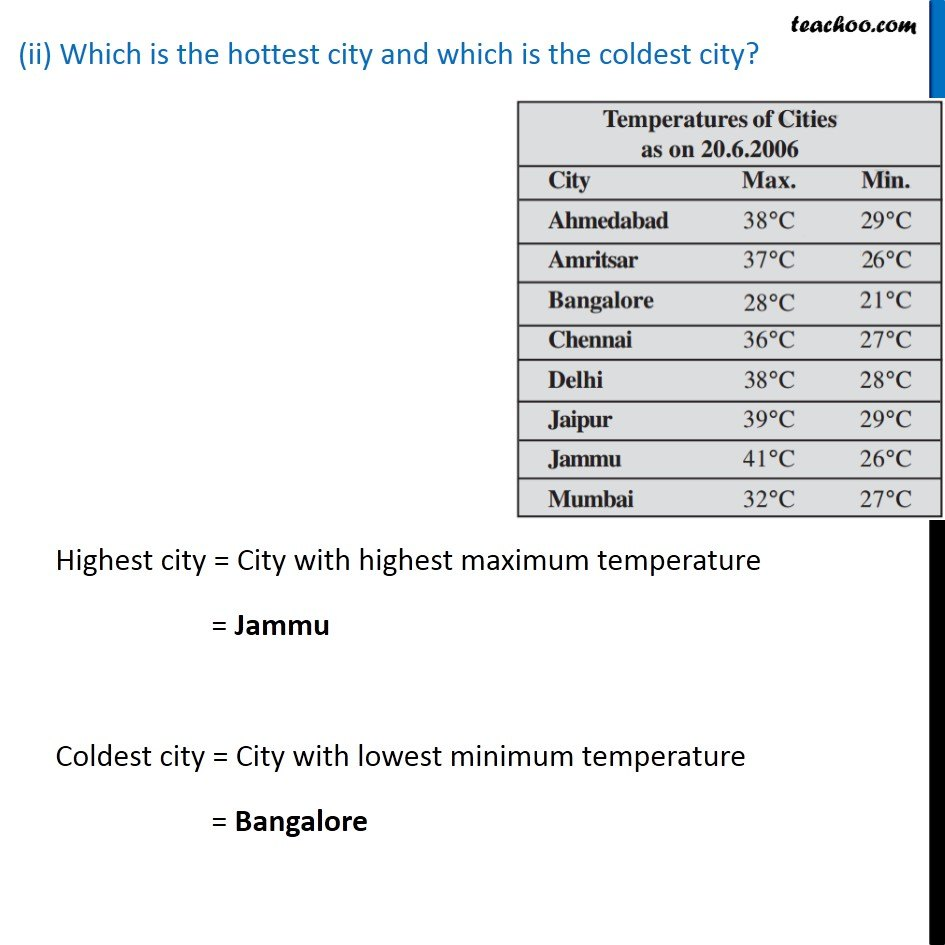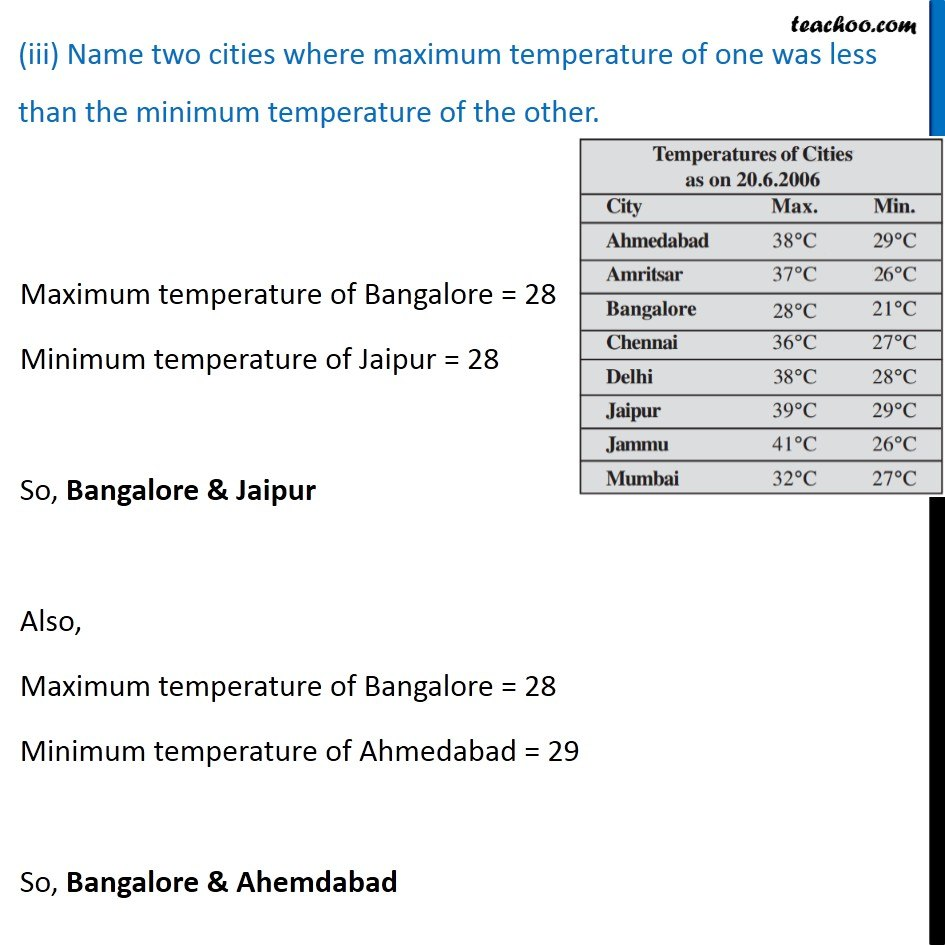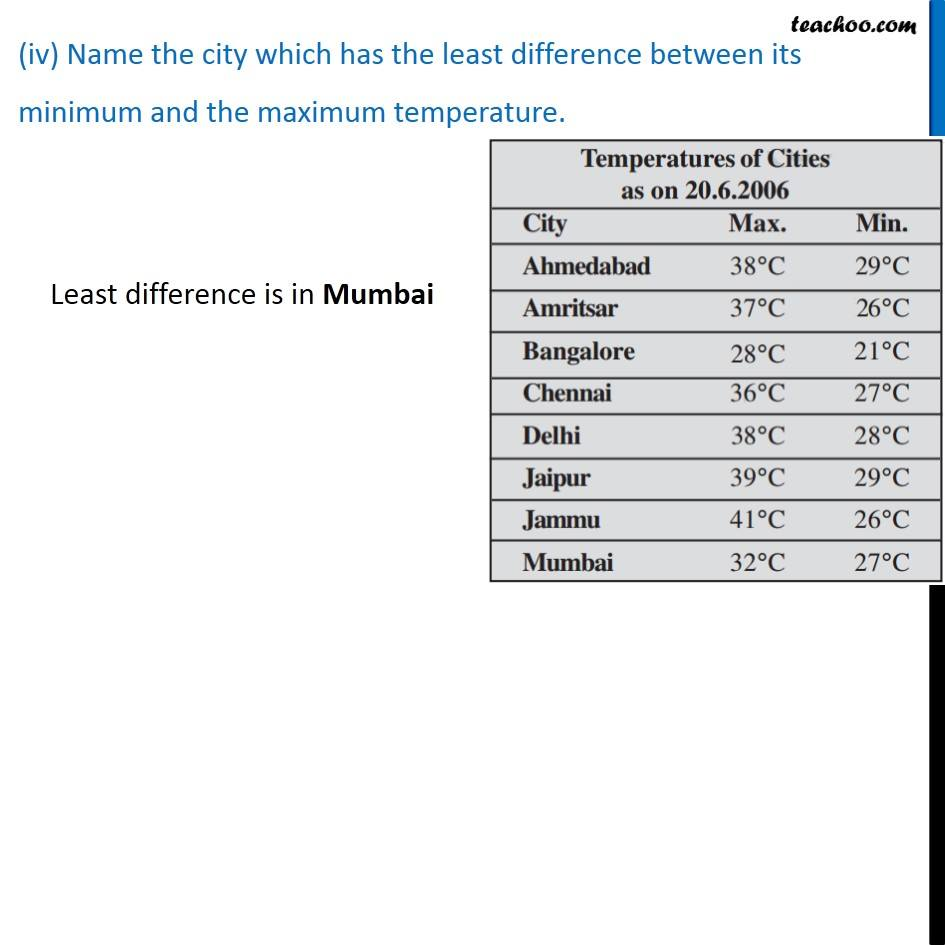1. Chapter 3 Class 7 Data Handling
2. Serial order wise
3. Ex 3.3

Transcript

Ex 3.3, 6 Take the data giving the minimum and the maximum temperature of various cities given in the beginning of this Chapter (Table 3.1). Plot a double bar graph using the data and answer the following: Here, temperature is from 21° C to 41° C So we draw a bar graph from 0 to 45 with a gap of 5 (i) Which city has the largest difference in the minimum and maximum temperature on the given date? Difference between minimum & maximum Temperature of Ahmedabad Temperature of Amritsar Temperature of Bangalore Temperature of Chennai Temperature of Delhi Temperature of Jaipur Temperature of Jammu Temperature of Mumbai So, largest temperature difference is in Jammu (ii) Which is the hottest city and which is the coldest city? Highest city = City with highest maximum temperature = Jammu Coldest city = City with lowest minimum temperature = Bangalore (iii) Name two cities where maximum temperature of one was less than the minimum temperature of the other. Maximum temperature of Bangalore = 28 Minimum temperature of Jaipur = 28 So, Bangalore & Jaipur Also, Maximum temperature of Bangalore = 28 Minimum temperature of Ahmedabad = 29 So, Bangalore & Ahemdabad (iv) Name the city which has the least difference between its minimum and the maximum temperature.

Ex 3.3